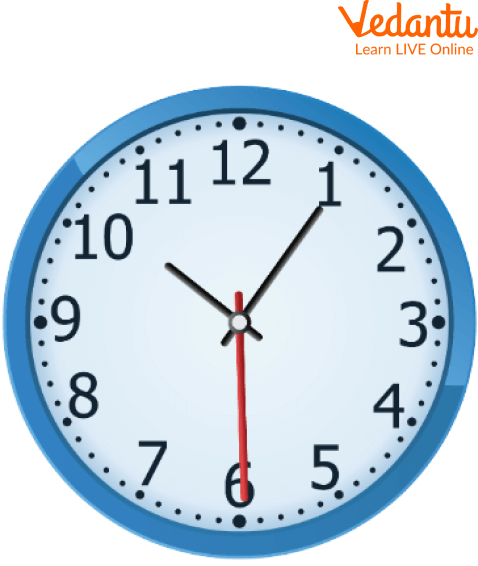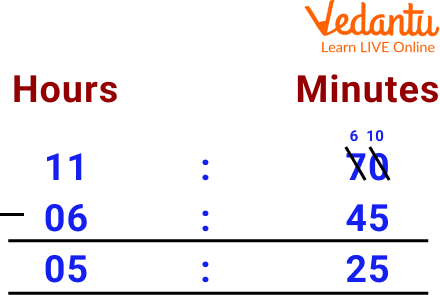Courses
Courses for Kids
Free study material
Offline Centres
More

# Addition and Subtraction of TimeLast updated date: 27th Nov 2023
Total views: 96.6k
Views today: 0.96k## Introduction to Addition and Subtraction of time

Time plays a crucial role in our lives. We use it to measure how long something takes, how much time we have left, and how much time is left before an event.

Time addition and subtraction are two math topics frequently taught in schools. There are many ways to teach these topics, but one way is using the clock face. Time is the most important factor in math. It can quickly and accurately calculate the time required to complete a task. There are many ways to do this, but one of the most efficient methods is adding and subtracting time.

This section will look at various methods for adding and subtracting time. We'll also talk about some common mistakes people make when calculating the amount of time it takes for a task or project.Clock

## Time and its Basic Unit

We can define time as a period during which a certain event happens, has happened or is going to happen. It is a measurable quantity and is also infinite. Time is measured in seconds, minutes, hours, days, months, and years. There are many other smaller units of time, such as milliseconds and microseconds. The smallest time unit is 'Zeptosecond'.

 Units of Time Value 1 day 24 hours 1 hour 60 minutes 1 minute 60 seconds

## How to Convert Between Hours, Minutes, and Seconds?

Conversion between hours, minutes, and seconds:

• $\text { Time in seconds }=\text { time in minutes } \times 60=\text { time in hours } \times 3600$

Convert 30 minutes into seconds.

We know that 1 minute $=60$ seconds,

So if we had to calculate how many seconds make 30 minutes,

We multiply 30 by 60,

Therefore, the total number of seconds in 30 minutes is 1800 seconds.

• $\text { Time in minutes }=\frac{\text { time in seconds }}{60}=\text { time in } \text { hours } \times 60$

Now, let us convert minutes and seconds into seconds for 40 minutes and 100 seconds.

We convert 40 minutes into seconds, $=40 \times 60$ seconds $=2400$ seconds.

$=2400+100 \text { seconds }=2500 \text { seconds }$

• $\text { Time in hours }=\frac{\text { time in minutes }}{60}=\frac{\text { time in } \text { seconds }}{3600}$

Now, let us convert hours and minutes into minutes

Let's take the example of 8 hours and 30 minutes

8 hours 30 minutes

$=(8 \times 60)+30 \text { minutes }=480+30 \text { minutes }=510 \text { minutes }$

Adding time in math is the process of adding distinct units of time such as hours, minutes, and seconds and then integrating them.

Adding time by hours and minutes is very simple if we know their conversion.

• First, add all the hours of the two times.

• Now add the minutes of the two given times.

• Further write the time obtained in hours: minutes format.

For example, let us add the set of two times, 9:10 and 1:15. Adding hours 9 and 1 equals 10. Further, adding minutes 10 and 15 is equal to 25. Further writing the calculated hours and minutes together, we have 10:25.

In some cases, if the added minutes are more than 60, we cannot take it directly. Instead, we must convert that into hours by subtracting the additional minutes.

Let us see this with another example:

Add 7:45 and 3:25. Here, we have 7 + 3 = 10, and 45 + 25 = 70. These 70 minutes cannot be taken directly. We know that 1 hour = 60 minutes. The 70 minutes can be written as 60 minutes + 10 minutes or 1 hour and 10 minutes. Therefore the time is 11 hours and 10 minutes or 11:10.

## Subtraction of Time

The subtraction of time is almost the same as the addition of time.

For this, we follow some simple steps.

• First, subtract the hours, and then subtract the minutes.

• Further, write the resultant answer of hours and minutes.

Let us look at this with a simple example. Let us subtract 5:45 from 7:55. First, let us subtract the hours 7 - 5 = 2; then, we can now subtract the minutes 55 - 45 = 10. The result can be written together as 2:10.

## Addition and Subtraction of Time Word Problems

Here, questions related to the word problem of addition and subtraction of time are given, which are like this;

Q 1. Add 38 minutes 40 seconds and 35 minutes 15 seconds.

Ans: First add the seconds 40 + 15 = 55 seconds

Second add the minutes, 38+35= 73 minutes

Convert, 73 minutes= 60+13= 1 hour 13 minutes

Because 1 hour = 60 minutes

 Minutes Seconds 38 40 35 15 73(1 hour 13 minutes) 55

Q 2. Add 26 minutes 35 seconds and 14 minutes 15 seconds.

Ans: First, add the seconds 35 + 15 = 50 seconds

Second, add the minutes, 26+14= 40 minutes

 Minutes Seconds 26 35 14 15 40 50

Q 3. Subtract 6:45 a.m. from 12:10 p.m.

Ans: We observe here that 45 minutes is more than 10 minutes. So, for carrying on subtraction, we need to borrow minutes from 12 hours and give to 10 minutes. By borrowing 60 minutes from 1 hour, we have 12 hours, 10 minutes is equal to 11 hours, 60 minutes + 10 minutes, or 11 hours and 70 minutes. Now, we have 70 minutes which is more than 45 minutes. So, we proceed with the following subtraction.Subtraction of time

Q 4. Subtract 42 minutes 55 seconds and 12 minutes 15 seconds.

Ans: First, add the seconds 55 - 15 = 40 seconds.

Second add the minutes, 42-12= 30 minutes

 Minutes Seconds 42 40 12 15 30 20

## Addition and Subtraction of Time Word Worksheet

Here Worksheet related to the word problem of addition and subtraction of time are given, which are like this;

Q 1. 40 minutes 15 seconds + 12 minutes 25 seconds.

Ans: 52 Minutes 40 Seconds

Q 2. Subtract 18 minutes 29 seconds from 24 minutes 15 seconds

Ans: 5 minutes 46 seconds

Q 3. Subtract 10 hours 50 minutes from 12 hours 45 minutes.

Ans: 1 hour 55 minutes

Q 4. Add 25 minutes 45 seconds and 15 minutes 25 seconds.

Ans: 41 minutes 10 seconds

5. 32 Minutes 15 Seconds - 12 Minutes and 5 Seconds

Ans. 20 Minutes 10 Seconds

## Summary

If you want to know how to add or subtract time in math, you will first need to know what the different units of measure are. The units of measure used for time are hours, minutes and seconds. The conversion table of time is a necessary tool for various mathematical calculations. Conversion of time either involves multiplication or division to change the unit. This means that you must multiply if you take a larger unit and convert it to a smaller one. But if you take a smaller unit and convert it to a larger one, you must divide it. There are two ways to add time in math: hours or minutes. To subtract time, we have to use the same procedure as subtracting it - by subtracting hours or minutes. This article will summarise the addition and subtraction of time in math.